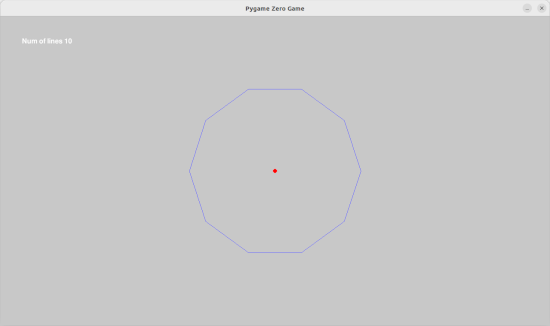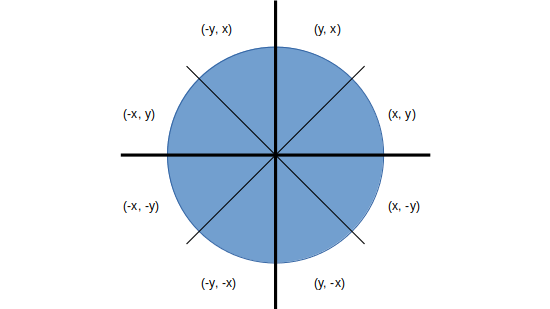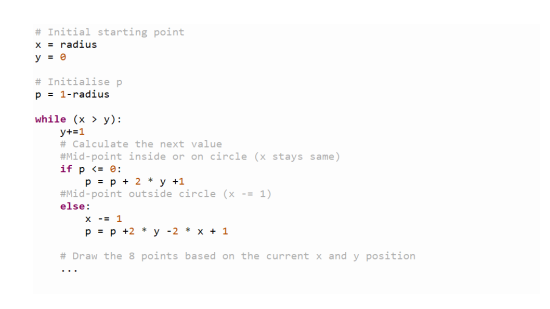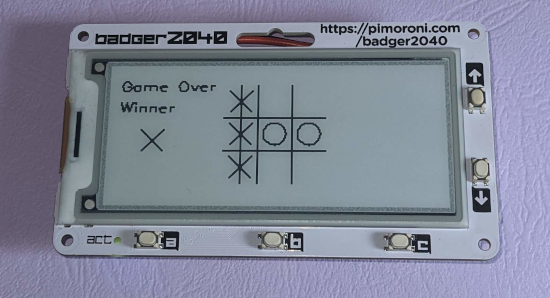# Computer Science - Beginners Algorithm Design - Circle Algorithm

This is an introduction to algorithm design. This is designed to be a simple explanation for those starting in computer science and watching to understand about why we design algorithms, how to create better algorithms and how to evaluate those algorithms.

This is based around a practical example of drawing circles on a Badger 2040 hackable ID badge, similar to the Raspberry Pi Pico.
This is going to explain about how I came up with 3 different algorithm designs. The first two I created using mathematical principles, the third is based on a real world algorithm which is more efficient.

In the video I also discuss some unique aspects of the Badger 2040 including how to create an interactive game which is usuable despite the refresh mechanism used for e-paper displays. This is based on a pass-and-play implementation of Tic-Tac-Toe.

## What is an algorithm?

An algoirithm is a process or set of rules which are followed to perform a calculation or to solve a problem. A useful analogy is with a cooking recipe. Just like a recipe describes the ingredients and how you combine them into something to eat, an algorithm tells you of the variables that you need and how the computer processes them to achieve the desired result. An algorithm can be as simple as saying increase x by 1, which would have something move left to right across the screen, or more complex such as finding the optimal route on a car satellite navigation system.

In this example the aim is to tell a computer how to draw a circle on the screen. This is done by calculating all the pixels that need to be coloured in. As you will see there are different ways that this can be achieved with different performance and correctness.

## Creating a circle using trigonometry

The first example is based on basic rules of trigonometry.The hypotenuse is shown as r, which is the radius of the circle. We need to know the co-ordinates x and y, which are calculated using the cosine and the sine of the angle. By starting the angle at zero and moving around the circle then the position of each of the pixels can be calculated.

An issue with this algorithm is that it involves a lot of processing to calculate all these points. This is known as time complexity.

## Creating a circle using straight lines (approximation algorithm)

The next algorithm is an approximation algorithm. The aim is to have an algorithm that creates an approximation of what is desired, or in this case something that looks like a circle, even if it’s not.

This is actually quite a common technique, especially for problems that are NP-hard, effectively those who would need huge amounts of processing to perform a certain task. An example is the classic travelling salesman problem which is about choosing an optimum route between cities. The idea of using an approximation algorithm is that rather than trying to calculate every possible solution to find the shortest (which you can do with a small number of cities) then to have an algorithm which instead works out a route that is approximately the best solution.

We also use approximations in other aspects of computer science. For example JPEG images you capture with a phone use a technique to reduce the size of the files by using a lossey compression algorithm. The aim to have a picture that looks the same to a human as the original, but uses much less storage.

The approximation I used with the circle is to have something that looks like it’s made with curved lines, but is actually made of straight lines. This is particularly useful for use with the Badger 2040 as the library has a function for drawing a straight line, but not one for drawing curved lines.

At it’s most accurate a line could be just 1 pixel long and it would be exactly the same as a true circle (in the case of my 200 pixel circle that’s made up of about 1000 lines), or we could reduce it to only 100 lines (a Hectogon) or 10 lines a Decagon (as shown in the image below).This is farly intuitive and easy to understand. It also reduces the number of trigonometry calculations needed. This is the technique I used in the tic-tac-toe game for the Badger 2040. The code for drawing the circle is shown below.

``````

degree_step_size = 10

# build list of points

points = []

for i in range (0, 360, degree_step_size):

points.append ((this_x, this_y))

for i in range(len(points)-1):

display.line (*points[i], *points[i+1])

# final back to the start

display.line(*points[len(points)-1], *points)

``````

## Mid-point Circle Drawing Algorithm

This is a more mathematically based algorithm which is used in real-world implementations of circle drawing functions.

For this to work you need to consider the circle split into 8 segments. The algorithm shown will calculate the values for the (x,y) segment and then you will need to apply the various translations to calculate for all 8 segments. This algorithm is based on calculating a single arc in the (x, y) segment.To implement this then you start at x = radius and y = 0
The next pixel is either (x, y+1) or (x-1, y+1).
To determine which then find the mid-point p of the two possible values (x-0.5, y+1) and if p is inside or on the perimeter then plot (x, y+1) otherwise plot (x-1, y+1).

To determine if p is inside or outside the circle use:
F(p) = x2 + y2 - r2

F(p) < 0 : inside the cricle
F(p) = 0 : on the perimeter
F(p) > 0 : outside the circle

The source code for the calculation of x, y and p is shown below.This has performance improvements compared to both the above and meets the correctness criteria.

## Tic-Tac-Toe Game

This has been used to create a game which you can play on the Badger 2040. It's a pass-and-play version of tic-tac-toe.This is the source code from the tic-tac-toe game. This uses the algorithrm that creates a circle using straight lines and the E-ink refresh settings.

``````

import time, math

# Global Constants

# Tic-Tac-Toe / Noughts and Crosses game

# Grid is x,y

#     x is 0 to 2 (left to right)

#     y is 0 to 2 (top to bottom)

def draw():

display.pen(15)

display.clear()

# Show grid

display.pen(0)

display.thickness(2)

display.line(103, 49, 193, 49)

display.line(103, 79, 193, 79)

display.line(133, 19, 133, 109)

display.line(163, 19, 163, 109)

# draw any played positions

for gy in range (0, 3):

for gx in range (0, 3):

if (grid[gx][gy] == 0):

# draw a cross

draw_cross(gx, gy)

elif (grid[gx][gy] == 1):

# draw a nought

draw_nought(gx, gy)

# Only show player and cursor if in game

if state == "play":

# Show current player

display.text("Player", 20, 20, 0.5)

if (player == 0):

draw_x (40, 50)

elif (player == 1):

draw_circle (40, 50)

draw_cursor(*cursor)

if state == "gameover":

display.text ("Game Over", 10, 20, 0.5)

# If there is a winner show the line

winner = is_won()

if winner >= 0:

# Draw line

display.line(*winning_line)

display.text ("Winner", 10, 40, 0.5)

if winner == 0:

draw_x (40, 70)

if winner == 1:

draw_circle (40, 70)

# It's a draw

else:

display.text ("Draw", 10, 40, 0.5)

# Draw the noughts and crosses

# Top level - draw nought on grid

def draw_nought(gridx, gridy):

# Approximation of a circle using lines

# get centre of circle

(cx, cy) = grid_to_coord(gridx, gridy)

draw_circle (cx, cy)

# Lower level needs co-ords - useful for player scores etc.

degree_step_size = 10

# build list of points

points = []

for i in range (0, 360, degree_step_size):

points.append ((this_x, this_y))

for i in range(len(points)-1):

display.line (*points[i], *points[i+1])

# final back to the start

display.line(*points[len(points)-1], *points)

# High level - draw cross on grid

def draw_cross(gridx, gridy):

# get centre of cross

(cx, cy) = grid_to_coord(gridx, gridy)

draw_x (cx, cy)

# Lower level needs co-ords - useful for player scores etc.

def draw_x (cx, cy):

display.line (cx -10, cy -10, cx + 10, cy + 10)

display.line (cx +10, cy -10, cx - 10, cy + 10)

def draw_cursor(gridx, gridy):

# draw square

(cx, cy) = grid_to_coord(gridx, gridy)

display.rectangle (cx -4, cy -4, 8, 8)

# ------------------------------

#        Helper methods

# ------------------------------

# Convert x,y position to centre of square

def grid_to_coord (gridx, gridy):

x = 118 + 30 * gridx

y = 34 + 30 * gridy

return (x, y)

# Checks for possible wins

def is_won ():

global willing_line

# start with first square on a possible line, check that has a player assigned

# then check rest of that line matches - if so then return the first square value

# which will equal the player number. If not return -1

# Check vertical lines

for col in range (0, 3):

if grid[col] != -1 and grid[col] == grid[col] and grid[col] == grid[col]:

winning_line = 118 + col * 30

winning_line = 19

winning_line = 118 + col * 30

winning_line = 109

return grid[col]

# Check horizontal lines

for line in range (0, 3):

if grid[line] != -1 and grid[line] == grid[line] and grid[line] == grid[line]:

winning_line = 103

winning_line = 34 + line * 30

winning_line = 193

winning_line = 34 + line * 30

return grid[line]

# check 2 diagnals

if grid != -1 and grid == grid and grid == grid:

winning_line = 103

winning_line = 19

winning_line = 193

winning_line = 109

return grid

if grid != -1 and grid == grid and grid == grid:

winning_line = 193

winning_line = 19

winning_line = 103

winning_line = 109

return grid

# reach here no match

return -1

# ------------------------------

#        Program setup

# ------------------------------

# Create a new Badger and set it to update NORMAL

display.led(128)

# ------------------------------

#       Main program

# ------------------------------

state = "play"

# Player 0 = crosses (goes first on first game)

# Player 1 = noughts

player = 0

cursor = [0,0]

# number of goes played - when reach 9 know that grid is full

played = 0

# Grid is x y (cols then rows) - so array does not look the same

# -1 = none

# 0 = player 0

# 1 = player 1

grid = [

[-1,-1, -1],

[-1,-1,-1],

[-1,-1,-1]]

# winning_line used as a global to determine where to draw line when someone wins

winning_line = [0,0,0,0]

# Display initial screen

draw()

display.update()

while (1):

# wait for action (uses loop)

while (1):

if state == "play":

if cursor > 0:

cursor -= 1

break

if cursor < 2:

cursor += 1

break

if cursor > 0:

cursor -= 1

break

if cursor < 2:

cursor += 1

break

# If empty then place the mark

# If not empty then ignore button press

if (grid[cursor][cursor] == -1):

grid[cursor][cursor] = player

played += 1

# Swap player and reset cursor

if player == 0:

player = 1

else:

player = 0

# return cursor to top left

cursor = 0

cursor = 0

break

# Otherwise press B to start game

else:

state == "play"

if is_won() >= 0 or played >= 9:

state = "gameover"

draw()

display.update()

``````